# Radiation Heat Transfer P M V Subbarao Associate

• Slides: 34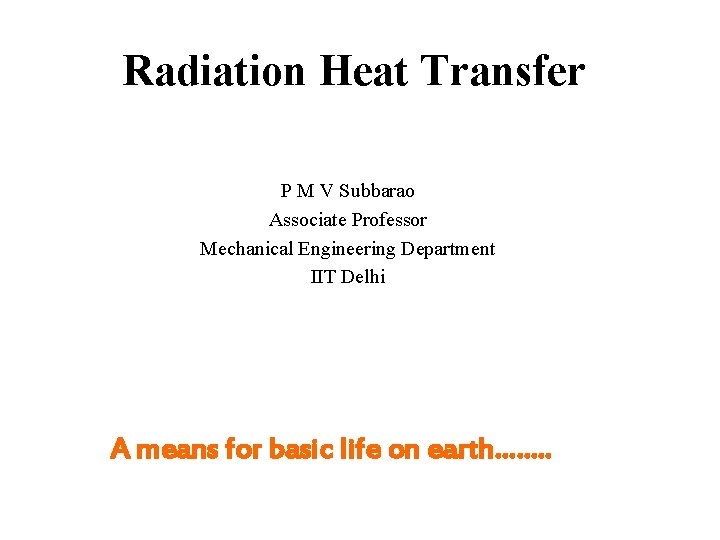Radiation Heat Transfer P M V Subbarao Associate Professor Mechanical Engineering Department IIT Delhi A means for basic life on earth……. .Introduction • Any matter with temperature above absolute zero (0 K) emits electromagnetic radiation. • In a simplified picture, radiation comes from the constantly changing electromagnetic fields of the oscillating atoms. • Electromagnetic radiation can be visualized as waves traveling at the speed of light. • The two prominent characters of the wave are the wavelength (λ) and frequency (ν). • The wavelength is the distance between crest to crest on the wave. • The frequency is related to wavelength by the following: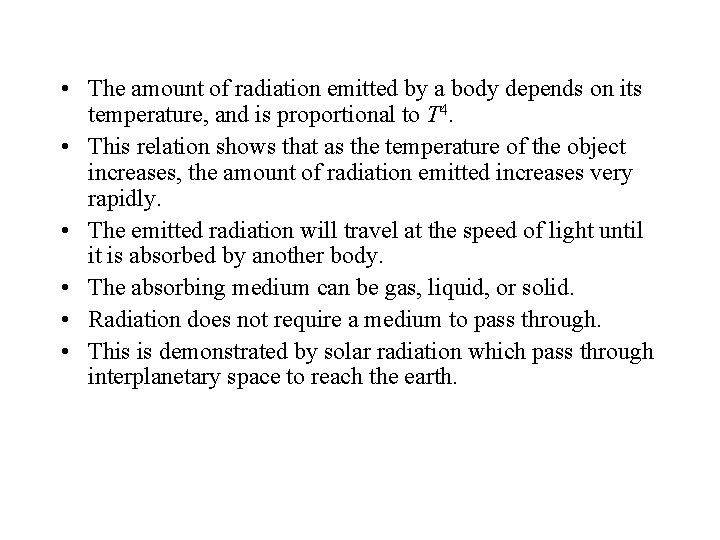• The amount of radiation emitted by a body depends on its temperature, and is proportional to T 4. • This relation shows that as the temperature of the object increases, the amount of radiation emitted increases very rapidly. • The emitted radiation will travel at the speed of light until it is absorbed by another body. • The absorbing medium can be gas, liquid, or solid. • Radiation does not require a medium to pass through. • This is demonstrated by solar radiation which pass through interplanetary space to reach the earth.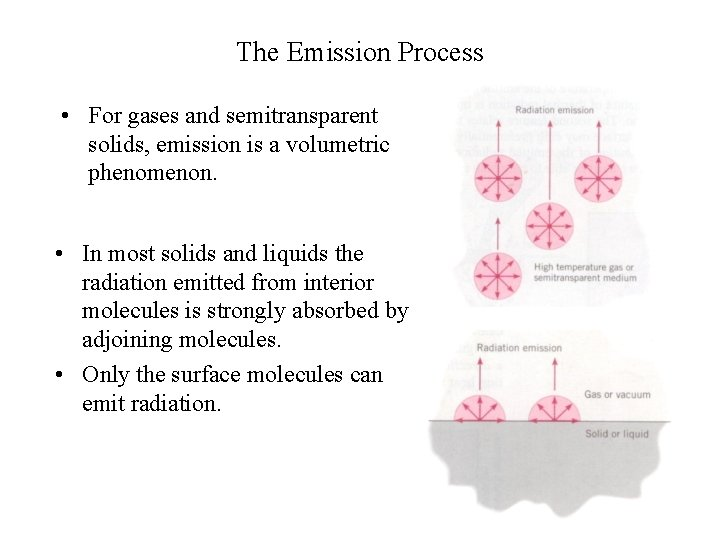The Emission Process • For gases and semitransparent solids, emission is a volumetric phenomenon. • In most solids and liquids the radiation emitted from interior molecules is strongly absorbed by adjoining molecules. • Only the surface molecules can emit radiation.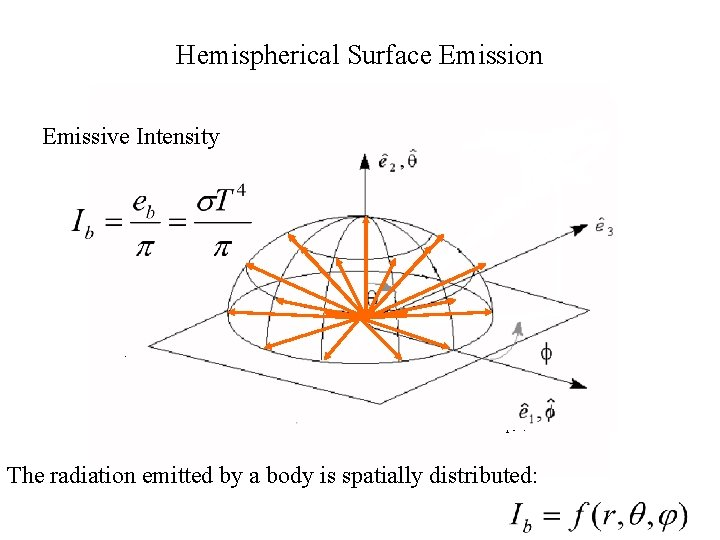Hemispherical Surface Emission Emissive Intensity The radiation emitted by a body is spatially distributed:Electromagnetic Spectrum • Electromagnetic radiation is categorized into types by their wavelengths. • The types of radiation and the respective wavelength ranges are shown in Figure. • Radiation with shorter wavelengths are more energetic, evident by the harmful gamma and xrays on the shorter end of the spectrum. • Radio waves, which are used to carry radio and TV signals, are much less energetic; however, they can pass through walls with no difficulty due to their long wavelengths. • The type of radiation emitted by a body depends on its temperature. • In general, the hotter the object is, the shorter the wavelengths of emitted radiation, and the greater the amount. • A much hotter body, such as the sun (~5800 K), emits the most radiation in the visible range.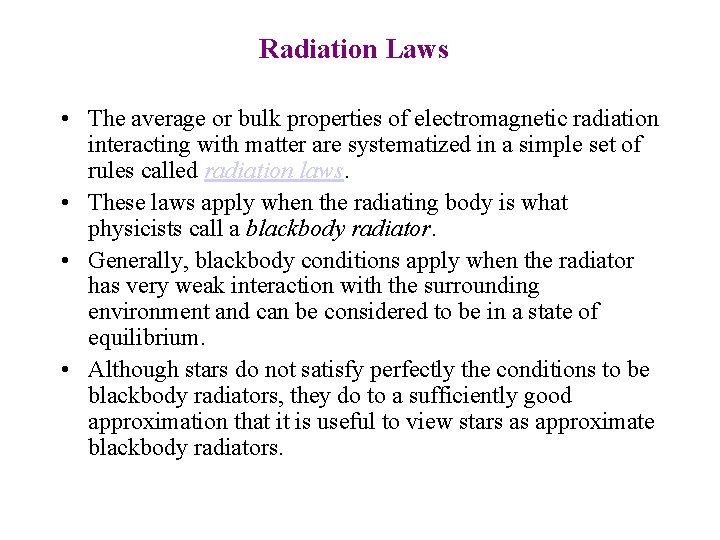Radiation Laws • The average or bulk properties of electromagnetic radiation interacting with matter are systematized in a simple set of rules called radiation laws. • These laws apply when the radiating body is what physicists call a blackbody radiator. • Generally, blackbody conditions apply when the radiator has very weak interaction with the surrounding environment and can be considered to be in a state of equilibrium. • Although stars do not satisfy perfectly the conditions to be blackbody radiators, they do to a sufficiently good approximation that it is useful to view stars as approximate blackbody radiators.Planck Radiation Law • The primary law governing blackbody radiation is the Planck Radiation Law. • This law governs the intensity of radiation emitted by unit surface area into a fixed direction (solid angle) from the blackbody as a function of wavelength for a fixed temperature. • The Planck Law can be expressed through the following equation. h = 6. 625 X 10 -27 erg-sec (Planck Constant) K = 1. 38 X 10 -16 erg/K (Boltzmann Constant) C = Speed of light in vacuum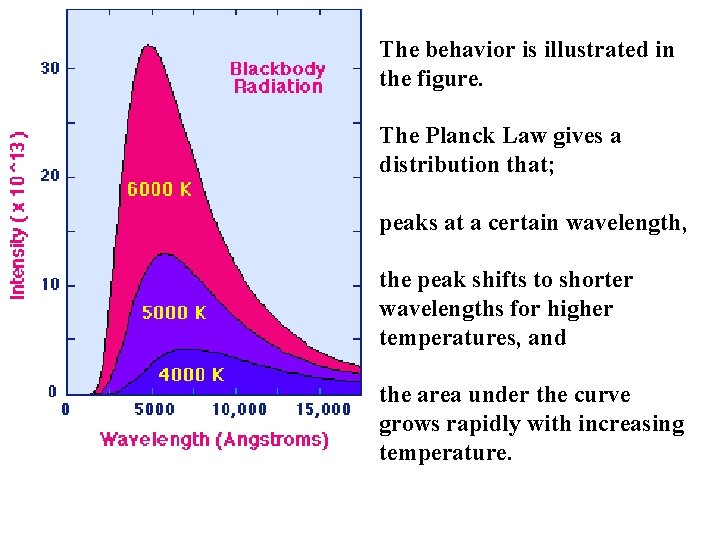The behavior is illustrated in the figure. The Planck Law gives a distribution that; peaks at a certain wavelength, the peak shifts to shorter wavelengths for higher temperatures, and the area under the curve grows rapidly with increasing temperature.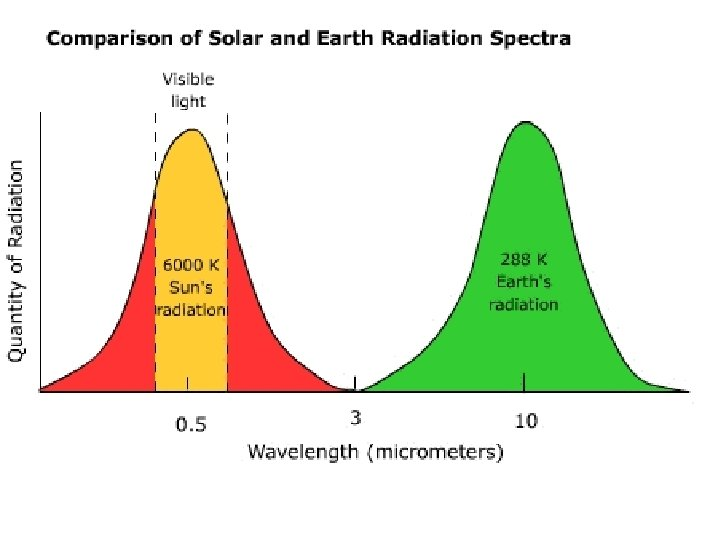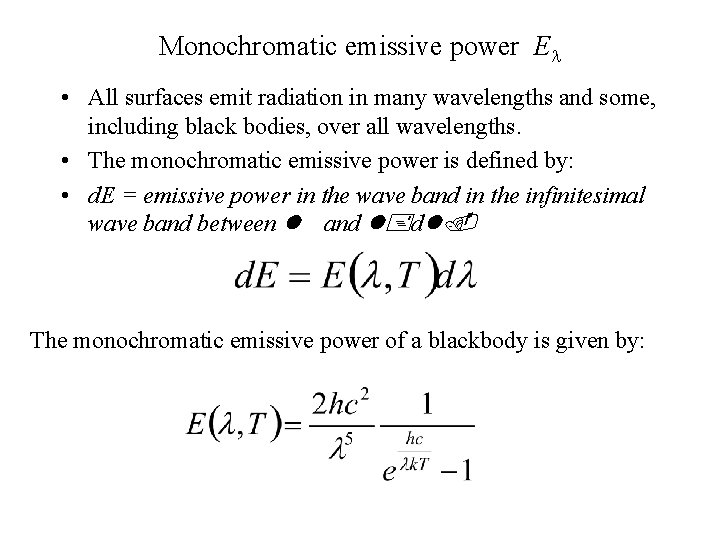Monochromatic emissive power El • All surfaces emit radiation in many wavelengths and some, including black bodies, over all wavelengths. • The monochromatic emissive power is defined by: • d. E = emissive power in the wave band in the infinitesimal wave band between l and l+dl. The monochromatic emissive power of a blackbody is given by: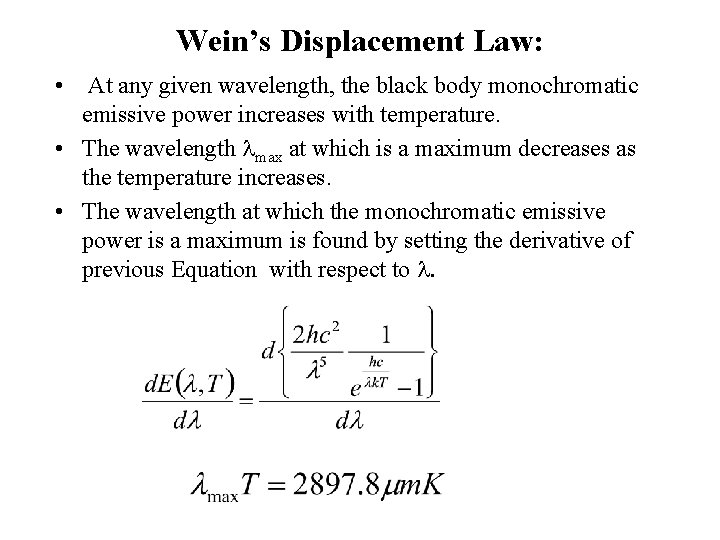Wein’s Displacement Law: • At any given wavelength, the black body monochromatic emissive power increases with temperature. • The wavelength lmax at which is a maximum decreases as the temperature increases. • The wavelength at which the monochromatic emissive power is a maximum is found by setting the derivative of previous Equation with respect to l.Wien law for three different stars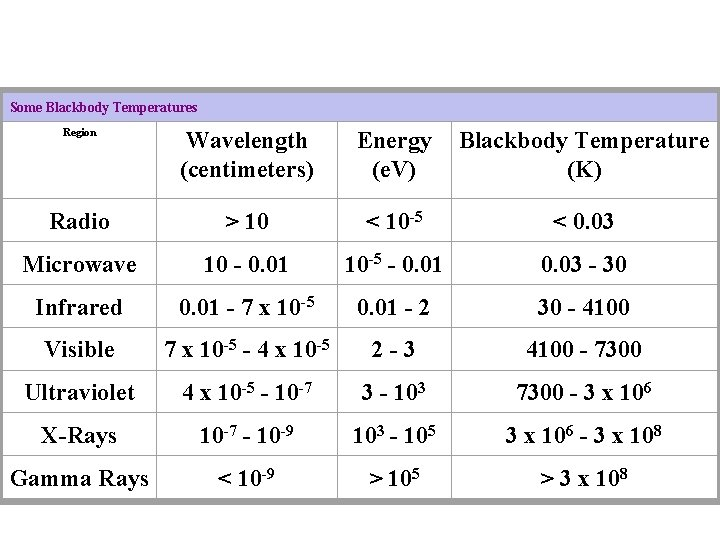Some Blackbody Temperatures Region Wavelength (centimeters) Energy (e. V) Blackbody Temperature (K) Radio > 10 < 10 -5 < 0. 03 Microwave 10 - 0. 01 10 -5 - 0. 01 0. 03 - 30 Infrared 0. 01 - 7 x 10 -5 0. 01 - 2 30 - 4100 Visible 7 x 10 -5 - 4 x 10 -5 2 -3 4100 - 7300 Ultraviolet 4 x 10 -5 - 10 -7 3 - 103 7300 - 3 x 106 X-Rays 10 -7 - 10 -9 103 - 105 3 x 106 - 3 x 108 Gamma Rays < 10 -9 > 105 > 3 x 108Stefan-Boltzmann Law • The maximum emissive power at a given temperature is the black body emissive power (Eb). • Integrating this over all wavelengths gives Eb.Relevance of Eb • Driving forces: Heat transfer by radiation is driven by differences in emissive power (proportional to T 4), not just temperature differences (convection & conduction).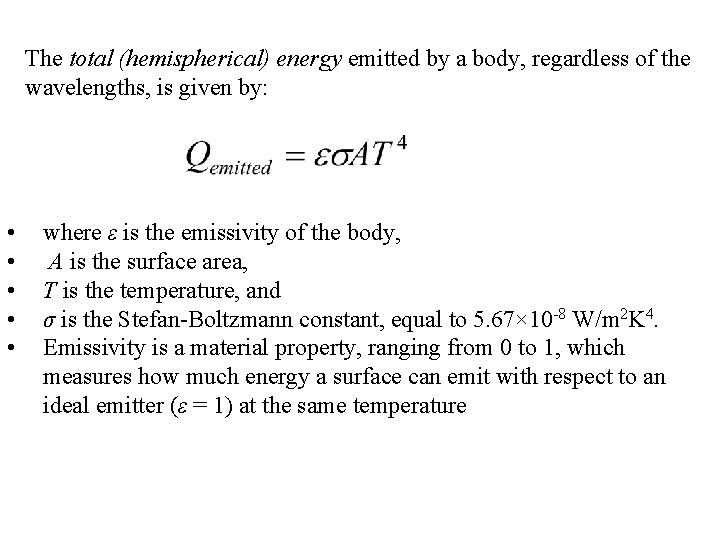The total (hemispherical) energy emitted by a body, regardless of the wavelengths, is given by: • • • where ε is the emissivity of the body, A is the surface area, T is the temperature, and σ is the Stefan-Boltzmann constant, equal to 5. 67× 10 -8 W/m 2 K 4. Emissivity is a material property, ranging from 0 to 1, which measures how much energy a surface can emit with respect to an ideal emitter (ε = 1) at the same temperature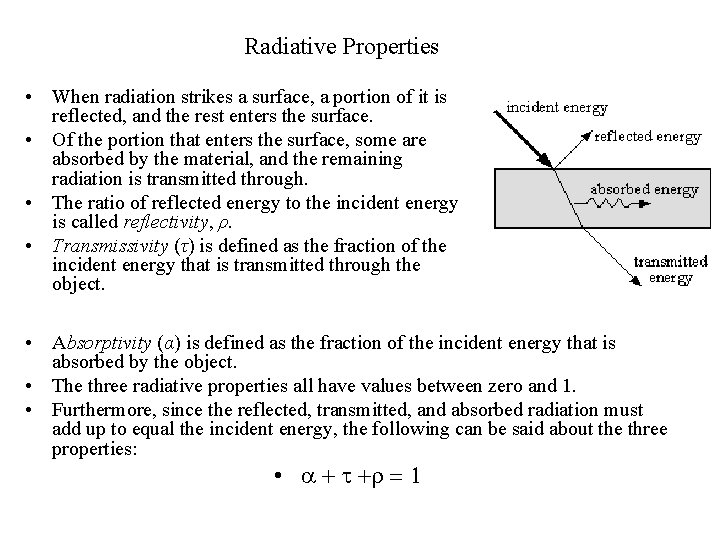Radiative Properties • When radiation strikes a surface, a portion of it is reflected, and the rest enters the surface. • Of the portion that enters the surface, some are absorbed by the material, and the remaining radiation is transmitted through. • The ratio of reflected energy to the incident energy is called reflectivity, ρ. • Transmissivity (τ) is defined as the fraction of the incident energy that is transmitted through the object. • Absorptivity (α) is defined as the fraction of the incident energy that is absorbed by the object. • The three radiative properties all have values between zero and 1. • Furthermore, since the reflected, transmitted, and absorbed radiation must add up to equal the incident energy, the following can be said about the three properties: • a + t +r = 1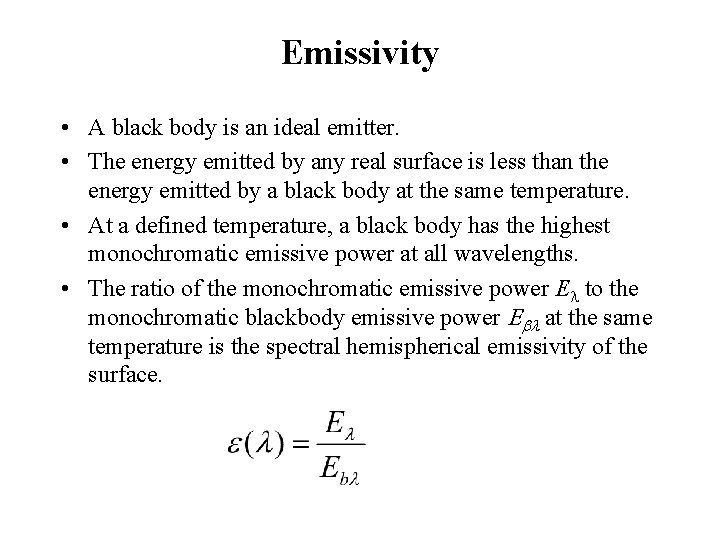Emissivity • A black body is an ideal emitter. • The energy emitted by any real surface is less than the energy emitted by a black body at the same temperature. • At a defined temperature, a black body has the highest monochromatic emissive power at all wavelengths. • The ratio of the monochromatic emissive power El to the monochromatic blackbody emissive power Ebl at the same temperature is the spectral hemispherical emissivity of the surface.The total (hemispherical emissive power is, then, given byë Define total (hemisherical) emissivity, at a defined temperature Here, e can be interpreted as either the emissivity of a body, which is wavelength independent, i. e. , el is constant, or as the average emissivity of a surface at that temperature. A surface whose properties are independent of the wavelength is known as a gray surface. The emissive power of a real surface is given by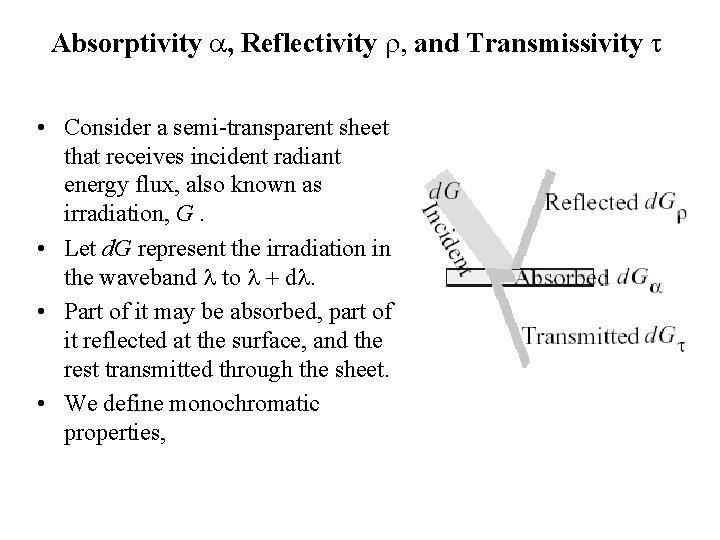Absorptivity a, Reflectivity r, and Transmissivity t • Consider a semi-transparent sheet that receives incident radiant energy flux, also known as irradiation, G. • Let d. G represent the irradiation in the waveband l to l + dl. • Part of it may be absorbed, part of it reflected at the surface, and the rest transmitted through the sheet. • We define monochromatic properties,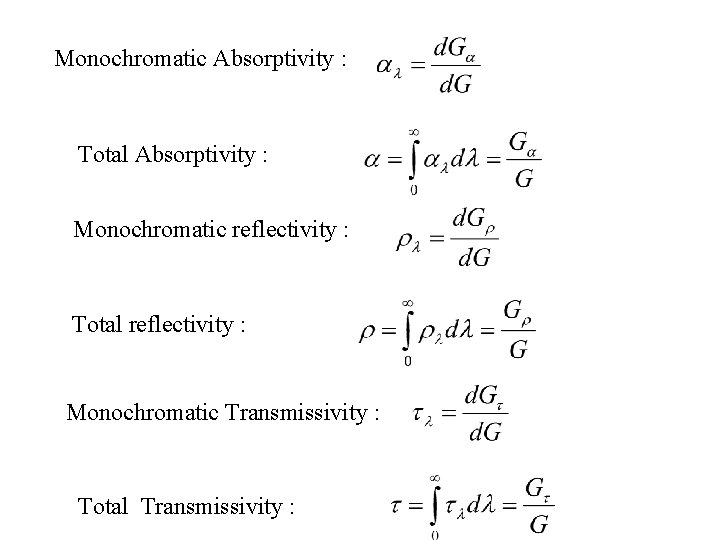Monochromatic Absorptivity : Total Absorptivity : Monochromatic reflectivity : Total reflectivity : Monochromatic Transmissivity : Total Transmissivity :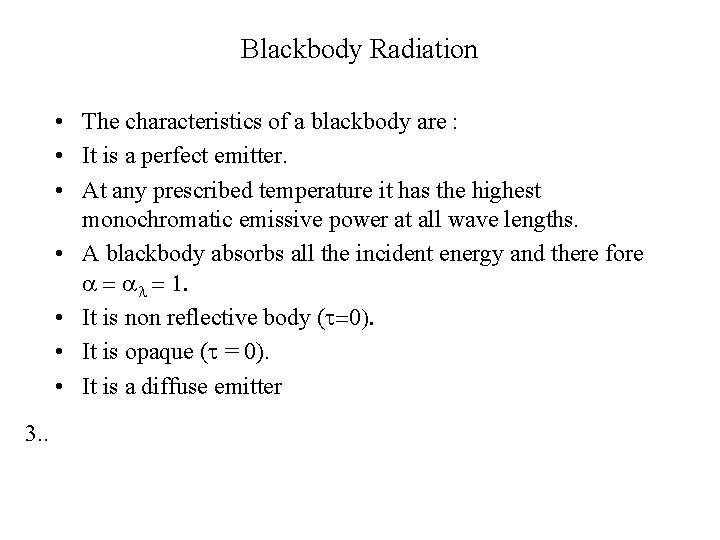Blackbody Radiation • The characteristics of a blackbody are : • It is a perfect emitter. • At any prescribed temperature it has the highest monochromatic emissive power at all wave lengths. • A blackbody absorbs all the incident energy and there fore a = al = 1. • It is non reflective body (t=0). • It is opaque (t = 0). • It is a diffuse emitter 3. .Radiative Heat Transfer Consider the heat transfer between two surfaces, as shown in Figure. What is the rate of heat transfer into Surface B? To find this, we will first look at the emission from A to B. Surface A emits radiation as described in This radiation is emitted in all directions, and only a fraction of it will actually strike Surface B. This fraction is called the shape factor, F.The amount of radiation striking Surface B is therefore: The only portion of the incident radiation contributing to heating Surface B is the absorbed portion, given by the absorptivity αB: Above equation is the amount of radiation gained by Surface B from Surface A. To find the net heat transfer rate at B, we must now subtract the amount of radiation emitted by B:The net radiative heat transfer (gain) rate at Surface B isShape Factors • Shape factor, F, is a geometrical factor which is determined by the shapes and relative locations of two surfaces. • Figure illustrates this for a simple case of cylindrical source and planar surface. • Both the cylinder and the plate are infinite in length. • In this case, it is easy to see that the shape factor is reduced as the distance between the source and plane increases. • The shape factor for this simple geometry is simply the cone angle (θ) divided by 2π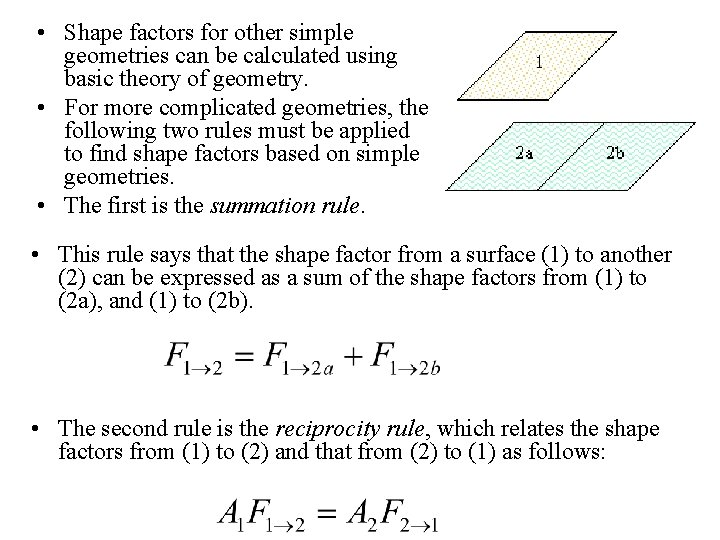• Shape factors for other simple geometries can be calculated using basic theory of geometry. • For more complicated geometries, the following two rules must be applied to find shape factors based on simple geometries. • The first is the summation rule. • This rule says that the shape factor from a surface (1) to another (2) can be expressed as a sum of the shape factors from (1) to (2 a), and (1) to (2 b). • The second rule is the reciprocity rule, which relates the shape factors from (1) to (2) and that from (2) to (1) as follows: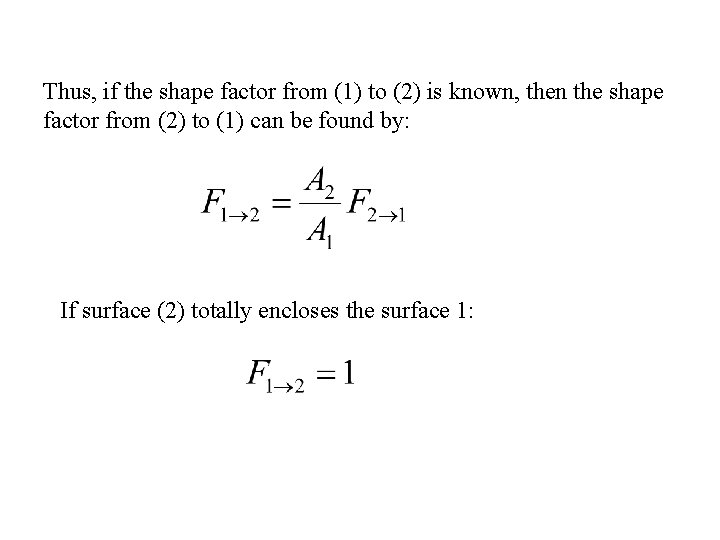Thus, if the shape factor from (1) to (2) is known, then the shape factor from (2) to (1) can be found by: If surface (2) totally encloses the surface 1:Geometric Concepts in Radiation • Solid Angle: Emissive intensity Monochromatic Emissive intensityTotal emissive power